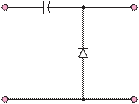### Create an Account

Home / Questions / Find the average value of the output voltage for the circuit of Figure P9 33 if the input ...

# Find the average value of the output voltage for the circuit of Figure P9 33 if the input voltage is sinusoidal with an amplitude of

Find the average value of the output voltage for the circuit of Figure P9.33 if the input voltage is sinusoidal with an amplitude of 5 V. Let Vγ = 0.7 V.

100 nF

to 100 and 500 Q.

9.30      A diode with the i-characteristic shown in

Figure 9.32 in the text is connected in series with a 2-V voltage source (in the forward bias direction) and a load resistance of 200 Q. Determine

a.    The load current and voltage.

b.    The power dissipated by the diode.

+vin

_

Figure P9.33

+

vout

_

to 100 and 300 Q.

Jun 18 2020 View more View LessSubscribe To Get Solution